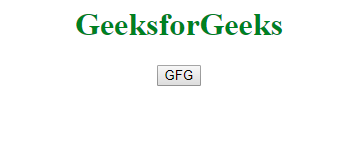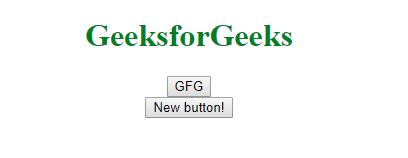Open in App
Not now

# How to create button dynamically with click event in Angular ?

• Last Updated : 07 Nov, 2019

The task is to create a button dynamically with click event using angular. In these examples when someone clicks on the button then a new button gets created.

The easiest way to create a button in AngularJS is to use the ng-repeat directive. We can easily hook up a repeat logic inside a button click event.

Syntax:

`<element ng-repeat="variable in expression"> Contents... </element>`

Example: Here we have a counter variable that keeps the count of buttons currently present in the DOM. it gets increased by one each time the main button (GFG) is pressed. the increased count results in a new button generated by ng-repeat.

 ` ` `<``html` `ng-app``=``"myApp"``> ` ` `  `<``head``> ` `    ``<``script` `src``= ` `"https://ajax.googleapis.com/ajax/libs/angularjs/1.7.8/angular.min.js"``> ` `    `` ` ` ` ` `  `<``body` `ng-controller``=``"MyController"``> ` `    ``<``center``> ` `        ``<``h1` `style``=``"color:green"``> ` `          ``GeeksforGeeks ` `      `` ` `        ``<``button` `type``=``"button"`  `                ``ng-click``=``"click(this)"``> ` `          ``GFG ` `      `` ` `        ``<``br``> ` `        ``` `        ``<``button` `type``=``"button"`  `                ``ng-repeat``=``"i in range(0, counter)"``> ` `          ``New button! ` `      `` ` `    `` ` ` ` `<``script` `type``=``"text/javascript"``> ` `    ``var myApp = angular.module('myApp', []); ` ` `  `    ``myApp.controller('MyController', ['\$scope', function(\$scope) { ` `        ``\$scope.counter = 0; ` ` `  `        ``\$scope.click = function(\$scope) { ` `            ``\$scope.counter++; ` `        ``} ` `        ``\$scope.range = ` `            ``function(min, max, step) { ` `                ``step = step || 1; ` `                ``var input = []; ` `                ``for (var i = min; i < ``max``; i += step) { ` `                    ``input.push(i); ` `                ``} ` `                ``return input; ` `            ``}; ` `    ``}]); ` ` ` ` `  ` `

Output: As we keep on clicking the button, the number of buttons keep on increasing in the DOM:My Personal Notes arrow_drop_up
Related Articles